# Engineering Mechanics - KOP: Force and Acceleration

## Why Engineering Mechanics KOP: Force and Acceleration?

In this section you can learn and practice Engineering Mechanics Questions based on "KOP: Force and Acceleration" and improve your skills in order to face the interview, competitive examination and various entrance test (CAT, GATE, GRE, MAT, Bank Exam, Railway Exam etc.) with full confidence.

## Where can I get Engineering Mechanics KOP: Force and Acceleration questions and answers with explanation?

IndiaBIX provides you lots of fully solved Engineering Mechanics (KOP: Force and Acceleration) questions and answers with Explanation. Solved examples with detailed answer description, explanation are given and it would be easy to understand. All students, freshers can download Engineering Mechanics KOP: Force and Acceleration quiz questions with answers as PDF files and eBooks.

## Where can I get Engineering Mechanics KOP: Force and Acceleration Interview Questions and Answers (objective type, multiple choice)?

Here you can find objective type Engineering Mechanics KOP: Force and Acceleration questions and answers for interview and entrance examination. Multiple choice and true or false type questions are also provided.

## How to solve Engineering Mechanics KOP: Force and Acceleration problems?

You can easily solve all kind of Engineering Mechanics questions based on KOP: Force and Acceleration by practicing the objective type exercises given below, also get shortcut methods to solve Engineering Mechanics KOP: Force and Acceleration problems.

### Exercise :: KOP: Force and Acceleration - General Questions

• KOP: Force and Acceleration - General Questions
1.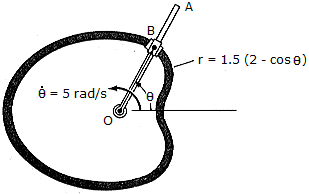Rod OA rotates counterclockwise with a constant angular rate of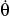= 5 rad/s. The double collar B is pin-connected together such that one collar slides over the rotating rod and the other slides over the horizontal curved rod, of which the shape is a limacon described by the equation r - 1.5(2 - cos) ft. If both collars weigh 0.75 lb, determine the normal force which the curved path exerts on one of the collars, and the force that OA exerts on the other collar at the instant= 90°.

 A. FOA = 0.873 lb, Fcurve = 1.953 lb B. FOA = 0, Fcurve = 1.953 lb C. FOA = 1.747 lb, Fcurve = 0 D. FOA = 2.87 lb, Fcurve = 6.41 lb

Explanation:

No answer description available for this question. Let us discuss.

2.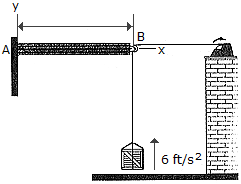The 30-lb crate is being hoisted upward with a constant acceleration of 6 ft/s2. If the uniform beam AB has a weight of 200 lb, determine the components of reaction at A. Neglect the size and mass of the pulley at B.

 A. Ax = -48.3 lb, Ay = 248.3 lb, MA = 258 lb-ft CCW B. Ax = -48.3 lb, Ay = 248.3 lb, MA = 741 lb-ft CCW C. Ax = -35.6 lb, Ay = 236 lb, MA = 678 lb-ft CCW D. Ax = -30.0 lb, Ay = 230 lb, MA = 650 lb-ft CCW

Explanation:

No answer description available for this question. Let us discuss.

3.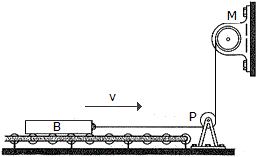The 300-kg bar B, originally at rest, is being towed over a series of small rollers. Computer the force in the cable when t = 5s, if the motor M is drawing in the cable for a short time at a rate of v = (0.4t2) m/s, where t is in seconds (0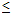t6 s). How far does the bar move in 5 s? Neglect the mass of the cable, pulley P, and the rollers.

 A. T = 5.00 kN, s = 0.300 m B. T = 1.200 kN, s = 1.25 m C. T = 5.00 kN, s = 4.00 m D. T = 1.200 kN, s = 16.67 m

Explanation:

No answer description available for this question. Let us discuss.

4.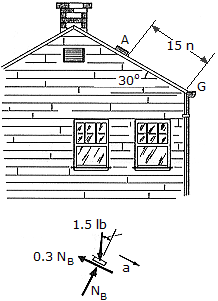A 1.5-lb brick is released from rest A and slides down the inclined roof. If the coefficient of friction between the roof and the brick is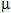= 0.3, determine the speed at which the brick strikes the gutter G.

 A. v = 3.00 ft/s B. v = 2.68 ft/s C. v = 5.61 ft/s D. v = 15.23 ft/s

Explanation:

No answer description available for this question. Let us discuss.

5.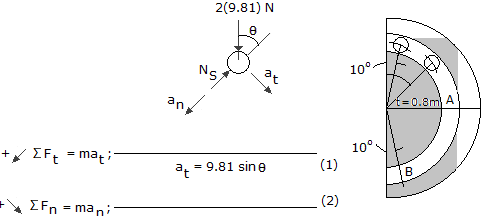A ball having a mass of 2 kg slides without friction within a vertical circular slot. If it is released from rest when= 10°, determine the force it exerts on the slot when it arrives at points A and B.

 A. NA = 38.6 N, NB = 96.6 N B. NA = 30.9 N, NB = 61.8 N C. NA = 30.9 N, NB = 81.2 N D. NA = 38.6 N, NB = 77.3 N